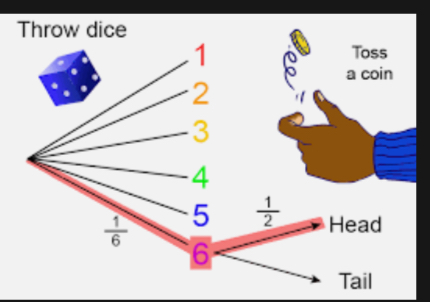I did not realize how the concept of probability could be applied in real situations. It always felt like another mathematical term but our class on Baye’s theorem was an eye opener. We analyzed cases using the knowledge of Baye’s theory. Bayes’ Rule lets you calculate the posterior probability. This is a conditional probability. It is the probability of the hypothesis being true, if the evidence is present

CASE:

The Wayne Manufacturing Company purchases a certain part from suppliers A, B and C. Supplier A supplies 60% of the parts, B 30% and C 10%. The quality of parts varies among the suppliers with A, B and C parts having 0.25%, 1% and 2% defective rates respectively.The parts are used in one of the company’s major products.

1. What is the probability that the company’s major product is assembled with a defective part? Use the tabular approach to Bayes’ theorem to solve.
2. When a defective part is found, which supplier is the likely source?

SOLUTION

P (D/A) = 0.0025

P (D/B) = 0.01

P (D/C) = 0.02

Where,

D= Prediction that the company’s major product is assembled with a defective part.

A = Prediction that the company’s major product is assembled with a defective part supplied by Supplier A.

B = Prediction that the company’s major product is assembled with a defective part supplied by Supplier B.

C = Prediction that the company’s major product is assembled with a defective part supplied by Supplier C

We will create the contingency table

From the table above,

P (D/A) = probability of the product being assembled with a defective part given the probability of A supplying the defective part.

P (D/B) = probability of the product being assembled with a defective part given the probability of B supplying the defective part.

P (D/C) = probability of the product being assembled with a defective part given the probability of C supplying the defective part.

P (D/S)= probability of the product being assembled with a defective part given the probability of the suppliers supplying the defective part.

P (D) = probability of the product being assembled with a defective part

P (A n D) = probability of the product being assembled with a defective part supplied by Supplier A

P (B n D) = probability of the product being assembled with a defective part supplied by Supplier B

P (C n D) = probability of the product being assembled with a defective part supplied by Supplier C

1. The probability that the company’s major product is assembled with a defective part is the sum of each supplier’s joint probability. The sum of P (D n A) + P (D n B) + P (D n C) i.e 0.0015 + 0.030+ 0.020 = 0.0065
• When a defective part is found, which supplier is the likely source? The like source is Supplier B because it has the highest posterior probability of 0.462 compared with Suppliers A and C, with posterior probability of 0.231 and 0.308 respectively.

LESSONS

On the surface value, we would have assumed that Supplier A is the likely source of a defective part because it supplies 60% of the total purchase. It is therefore very important to have full understanding and other data to aid proper analysis that will help us take great decisions.

#MMBA3## Understanding the Balance Sheet and the Income Statement: A Guide for Business Managers #MMBA5

in